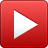# John Queen

## John Queen

•#### Growing Up in Poverty /* <![CDATA[ */ jQuery(document).ready(function(\$){ \$(function(){ var width = \$(window).innerWidth(); var setwidth = parseFloat(640); var ratio = parseFloat(0.75); var height = parseFloat(480); var link = 'https://www.youtube.com/watch?v=3yLoO1jO9Ls&amp;width=640&amp;height=480'; if(width < setwidth) { height = Math.floor(width * 0.75); //console.log("device width "+width+", set width "+640+", ratio "+0.75+", new height "+ height); var new_url = wpvl_paramReplace('width', link, width); var new_url = wpvl_paramReplace('height', new_url, height); \$("a#647fe072dcabe").attr('href', new_url); //console.log(new_url); } }); }); /* ]]> */

•#### HOYAS /* <![CDATA[ */ jQuery(document).ready(function(\$){ \$(function(){ var width = \$(window).innerWidth(); var setwidth = parseFloat(640); var ratio = parseFloat(0.75); var height = parseFloat(480); var link = 'https://www.youtube.com/watch?v=3sbeeKbPz2M&amp;width=640&amp;height=480'; if(width < setwidth) { height = Math.floor(width * 0.75); //console.log("device width "+width+", set width "+640+", ratio "+0.75+", new height "+ height); var new_url = wpvl_paramReplace('width', link, width); var new_url = wpvl_paramReplace('height', new_url, height); \$("a#647fe072dcb28").attr('href', new_url); //console.log(new_url); } }); }); /* ]]> */

•#### Need Male Role Models /* <![CDATA[ */ jQuery(document).ready(function(\$){ \$(function(){ var width = \$(window).innerWidth(); var setwidth = parseFloat(640); var ratio = parseFloat(0.75); var height = parseFloat(480); var link = 'https://www.youtube.com/watch?v=3BrPVIoXepU&amp;width=640&amp;height=480'; if(width < setwidth) { height = Math.floor(width * 0.75); //console.log("device width "+width+", set width "+640+", ratio "+0.75+", new height "+ height); var new_url = wpvl_paramReplace('width', link, width); var new_url = wpvl_paramReplace('height', new_url, height); \$("a#647fe072dcb7c").attr('href', new_url); //console.log(new_url); } }); }); /* ]]> */

•#### Challenges Our Youth Face /* <![CDATA[ */ jQuery(document).ready(function(\$){ \$(function(){ var width = \$(window).innerWidth(); var setwidth = parseFloat(640); var ratio = parseFloat(0.75); var height = parseFloat(480); var link = 'https://www.youtube.com/watch?v=MScvFhXjqYo&amp;width=640&amp;height=480'; if(width < setwidth) { height = Math.floor(width * 0.75); //console.log("device width "+width+", set width "+640+", ratio "+0.75+", new height "+ height); var new_url = wpvl_paramReplace('width', link, width); var new_url = wpvl_paramReplace('height', new_url, height); \$("a#647fe072dcbcd").attr('href', new_url); //console.log(new_url); } }); }); /* ]]> */

•#### Why Kent County? /* <![CDATA[ */ jQuery(document).ready(function(\$){ \$(function(){ var width = \$(window).innerWidth(); var setwidth = parseFloat(640); var ratio = parseFloat(0.75); var height = parseFloat(480); var link = 'https://www.youtube.com/watch?v=WOv-N6UL14o&amp;width=640&amp;height=480'; if(width < setwidth) { height = Math.floor(width * 0.75); //console.log("device width "+width+", set width "+640+", ratio "+0.75+", new height "+ height); var new_url = wpvl_paramReplace('width', link, width); var new_url = wpvl_paramReplace('height', new_url, height); \$("a#647fe072dcc25").attr('href', new_url); //console.log(new_url); } }); }); /* ]]> */

•#### Caring Goes a Long Way /* <![CDATA[ */ jQuery(document).ready(function(\$){ \$(function(){ var width = \$(window).innerWidth(); var setwidth = parseFloat(640); var ratio = parseFloat(0.75); var height = parseFloat(480); var link = 'https://www.youtube.com/watch?v=0LVw2uxiiBM&amp;width=640&amp;height=480'; if(width < setwidth) { height = Math.floor(width * 0.75); //console.log("device width "+width+", set width "+640+", ratio "+0.75+", new height "+ height); var new_url = wpvl_paramReplace('width', link, width); var new_url = wpvl_paramReplace('height', new_url, height); \$("a#647fe072dcc75").attr('href', new_url); //console.log(new_url); } }); }); /* ]]> */

•#### Learning From My Wife /* <![CDATA[ */ jQuery(document).ready(function(\$){ \$(function(){ var width = \$(window).innerWidth(); var setwidth = parseFloat(640); var ratio = parseFloat(0.75); var height = parseFloat(480); var link = 'https://www.youtube.com/watch?v=sXhWSvbiKrQ&amp;width=640&amp;height=480'; if(width < setwidth) { height = Math.floor(width * 0.75); //console.log("device width "+width+", set width "+640+", ratio "+0.75+", new height "+ height); var new_url = wpvl_paramReplace('width', link, width); var new_url = wpvl_paramReplace('height', new_url, height); \$("a#647fe072dccc7").attr('href', new_url); //console.log(new_url); } }); }); /* ]]> */

•#### Measuring Success /* <![CDATA[ */ jQuery(document).ready(function(\$){ \$(function(){ var width = \$(window).innerWidth(); var setwidth = parseFloat(640); var ratio = parseFloat(0.75); var height = parseFloat(480); var link = 'https://www.youtube.com/watch?v=d5CjHvx_wis&amp;width=640&amp;height=480'; if(width < setwidth) { height = Math.floor(width * 0.75); //console.log("device width "+width+", set width "+640+", ratio "+0.75+", new height "+ height); var new_url = wpvl_paramReplace('width', link, width); var new_url = wpvl_paramReplace('height', new_url, height); \$("a#647fe072dcd91").attr('href', new_url); //console.log(new_url); } }); }); /* ]]> */

•#### Giving 100% /* <![CDATA[ */ jQuery(document).ready(function(\$){ \$(function(){ var width = \$(window).innerWidth(); var setwidth = parseFloat(640); var ratio = parseFloat(0.75); var height = parseFloat(480); var link = 'https://www.youtube.com/watch?v=xRRmivypGWc&amp;width=640&amp;height=480'; if(width < setwidth) { height = Math.floor(width * 0.75); //console.log("device width "+width+", set width "+640+", ratio "+0.75+", new height "+ height); var new_url = wpvl_paramReplace('width', link, width); var new_url = wpvl_paramReplace('height', new_url, height); \$("a#647fe072dcde1").attr('href', new_url); //console.log(new_url); } }); }); /* ]]> */

•#### Balancing Family and HOYAS /* <![CDATA[ */ jQuery(document).ready(function(\$){ \$(function(){ var width = \$(window).innerWidth(); var setwidth = parseFloat(640); var ratio = parseFloat(0.75); var height = parseFloat(480); var link = 'https://www.youtube.com/watch?v=LOuXF6A9Ss0&amp;width=640&amp;height=480'; if(width < setwidth) { height = Math.floor(width * 0.75); //console.log("device width "+width+", set width "+640+", ratio "+0.75+", new height "+ height); var new_url = wpvl_paramReplace('width', link, width); var new_url = wpvl_paramReplace('height', new_url, height); \$("a#647fe072dce36").attr('href', new_url); //console.log(new_url); } }); }); /* ]]> */

Interview and video production by Lani Seikaly